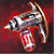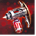# Ancient CorruptionConditions

## Related effects, buffs and debuffs

• [hidden] [does not expire]Effect #1

 Slot: Buff Duration: passive Tick rate: does not tick # occurrences: 0
• On Apply

Perform the following actions:

• Unknown (183)
- Unknown (303) = (bool) true
- Unknown (306) = (bool) true
- Unknown (584) = (bool) false
- Unknown (310) = (string) aoePositionOptionType_WorldCoordinates
- Unknown (313) = (string) aoeScalarType_LiteralValue
- Unknown (317) = (string) aoeScalarType_LiteralValue
- Unknown (321) = (string) aoeScalarType_LiteralValue
- Unknown (325) = (string) aoeOrientationOptionType_WorldCoordinates
- Unknown (344) = (string) aoeSphere
- Unknown (349) = (string) aoeTargetCondition_IsPlayer;aoeTargetCondition_IsAlive
- Unknown (353) = (string) aoeScalarType_LiteralValue
- Unknown (354) = (string) aoeScalarType_LiteralValue
- Unknown (355) = (string) aoeScalarType_LiteralValue
- Unknown (367) = (string) aoeScalarType_LiteralValue
- Unknown (384) = (string)
- Unknown (386) = (string) aoeAbilityCaster_Caster
- Unknown (387) = (string) aoeAbilityTarget_Target
- Unknown (388) = (string) worlddesign/exp/03/onderon/datacron/death_fog_aoe_sphere
- Unknown (389) = (string)
- Unknown (431) = (string) aoeRotation_None
- Unknown (534) = (string) aoeScaling_FixedAmount
- Unknown (541) = (string) aoeVfxEntity_AreaEffect
- Unknown (542) = (string)
- Unknown (551) = (string) aoeVfx_PlayAtPosition
- Unknown (552) = (string) aoeVfx_None
- Unknown (587) = (string) aoeScaling_StopScaling
- Effect Number = (int) 0
- Ability Spec = (int) 16141133552376187870
- Unknown (307) = (int) 1
- Unknown (308) = (int) 3600
- Tick Rate = (float) 1
- Unknown (304) = (float) 3600
- Unknown (305) = (float) 3600
- Unknown (314) = (float) -6.924
- Unknown (318) = (float) 7.78
- Unknown (322) = (float) -107.89
- Unknown (362) = (float) 0
- Unknown (363) = (float) 0
- Unknown (364) = (float) 0
- Unknown (368) = (float) 0.1
- Unknown (390) = (float) 0
- Unknown (398) = (float) 0.1
- Unknown (582) = (float) 20
- Unknown (592) = (float) 0.3333
- Unknown (616) = (float) 1
- Unknown (617) = (float) 1
- Unknown (618) = (float) 1
- Unknown (623) = (float) 1
- Unknown (624) = (float) 1
- Unknown (625) = (float) 1
• Just before this effect is removed

Perform the following actions:

• Execute Hydra Hook
- Event Name = (string) Explosion_cleanup
- Object Self = (int) 1